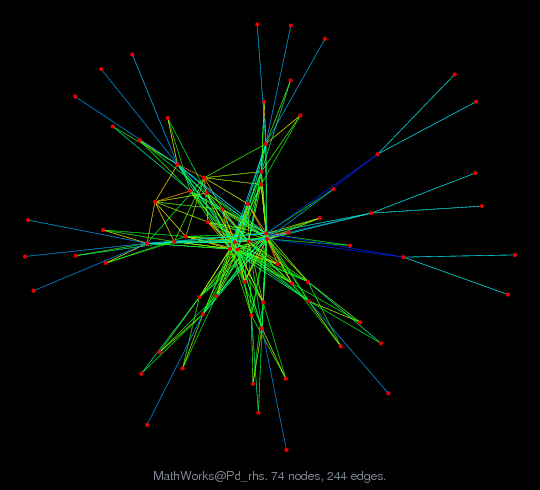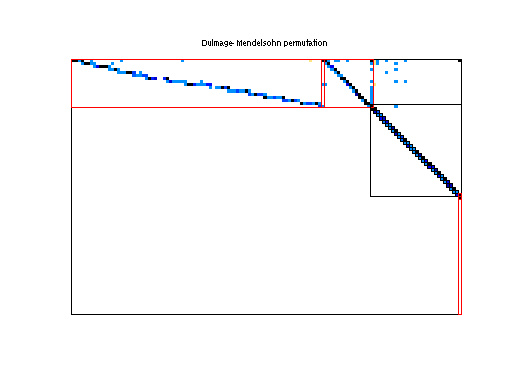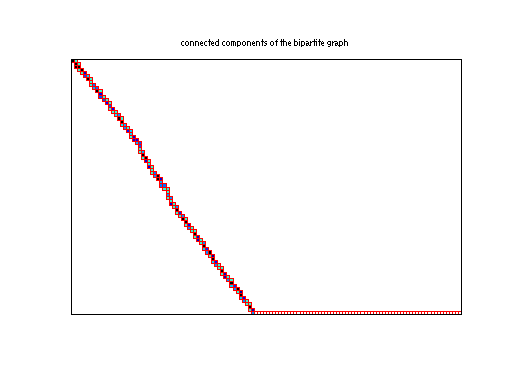Matrix: MathWorks/Pd_rhs

Description: Matrix problem X=A\B with many sparse right-hand-sides(bipartite graph drawing)• Matrix group: MathWorks
• download as a MATLAB mat-file, file size: 25 KB. Use UFget(1171) or UFget('MathWorks/Pd_rhs') in MATLAB.

 Matrix properties number of rows 8,081 number of columns 12,406 nonzeros 6,323 structural full rank? no structural rank 4,368 # of blocks from dmperm 2,872 # strongly connected comp. 14,549 explicit zero entries 0 nonzero pattern symmetry 0% numeric value symmetry 0% type real structure rectangular Cholesky candidate? no positive definite? no

 author P. Anderson editor T. Davis date 2002 kind counter-example problem 2D/3D problem? no

 Ordering statistics: result nnz(V) for QR, upper bound nnz(L) for LU, with COLAMD 9,829 nnz(R) for QR, upper bound nnz(U) for LU, with COLAMD 8,788

 SVD-based statistics: norm(A) 135541 min(svd(A)) 0 cond(A) Inf rank(A) 4,368 sprank(A)-rank(A) 0 null space dimension 3,713 full numerical rank? no singular value gap 6.73494e+07

 singular values (MAT file): click here SVD method used: s = svd (full (A)) ; status: ok